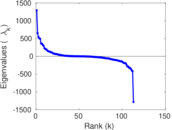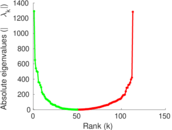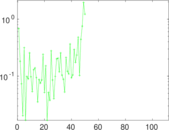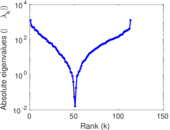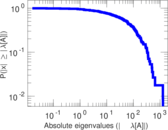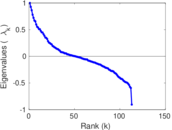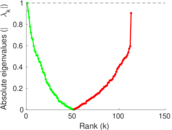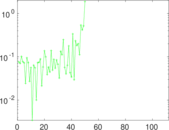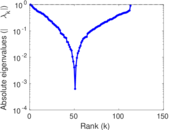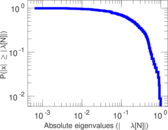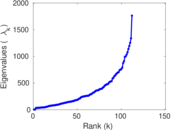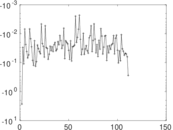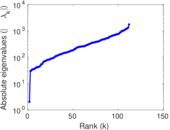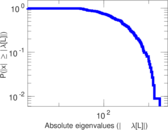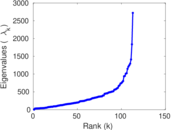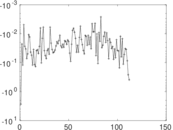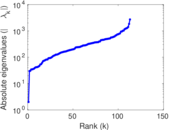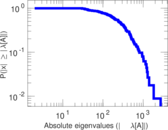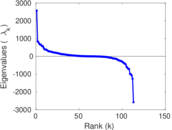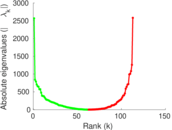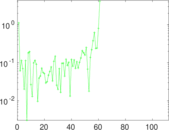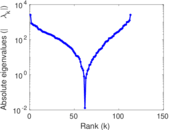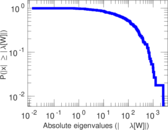# Hypertext 2009

This is the network of face-to-face contacts of the attendees of the ACM Hypertext 2009 conference. The ACM Conference on Hypertext and Hypermedia 2009 (HT 2009, http://www.ht2009.org/) was held in Turin, Italy over three days from June 29 to July 1, 2009. In the network, a node represents a conference visitor, and an edge represents a face-to-face contact that was active for at least 20 seconds. Multiple edges denote multiple contacts. Each edge is annotated with the time at which the contact took place.

 Code `HY` Internal name `sociopatterns-hypertext` Name Hypertext 2009 Data source http://www.sociopatterns.org/ AvailabilityDataset is available for download Consistency checkDataset passed all tests Category Human contact network Dataset timestamp 2009-06-29 ⋯ 2009-07-01 Node meaning Visitor Edge meaning Contact Network format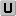Unipartite, undirected Edge typeUnweighted, multiple edges Temporal dataEdges are annotated with timestamps LoopsDoes not contain loops

## Statistics

 Size n = 113 Volume m = 20,818 Unique edge count m̿ = 2,196 Loop count l = 0 Wedge count s = 102,182 Claw count z = 1,810,498 Cross count x = 26,678,148 Triangle count t = 16,867 Square count q = 558,736 4-Tour count T4 = 4,883,008 Maximum degree dmax = 1,483 Average degree d = 368.460 Fill p = 0.347 029 Average edge multiplicity m̃ = 9.479 96 Size of LCC N = 113 Diameter δ = 3 50-Percentile effective diameter δ0.5 = 1.160 76 90-Percentile effective diameter δ0.9 = 1.841 12 Median distance δM = 2 Mean distance δm = 1.591 31 Gini coefficient G = 0.457 576 Balanced inequality ratio P = 0.328 514 Relative edge distribution entropy Her = 0.976 022 Power law exponent γ = 1.283 93 Tail power law exponent γt = 4.171 00 Tail power law exponent with p γ3 = 4.171 00 p-value p = 0.001 000 00 Degree assortativity ρ = −0.122 580 Degree assortativity p-value pρ = 3.576 18 × 10−16 Clustering coefficient c = 0.495 205 Spectral norm α = 1,291.72 Algebraic connectivity a = 2.013 10 Spectral separation |λ1[A] / λ2[A]| = 1.006 03 Non-bipartivity bA = 0.005 997 03 Normalized non-bipartivity bN = 0.764 945 Algebraic non-bipartivity χ = 0.989 464 Spectral bipartite frustration bK = 0.006 364 38 Controllability C = 0 Relative controllability Cr = 0.000 00

## Plots

### Fruchterman–Reingold graph drawing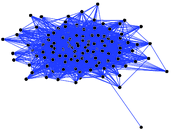### Degree distribution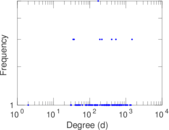### Cumulative degree distribution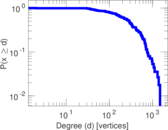### Lorenz curve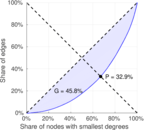### Spectral distribution of the adjacency matrix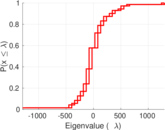### Spectral distribution of the normalized adjacency matrix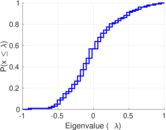### Spectral distribution of the Laplacian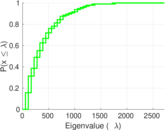### Spectral graph drawing based on the adjacency matrix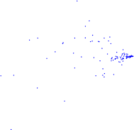### Spectral graph drawing based on the Laplacian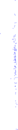### Spectral graph drawing based on the normalized adjacency matrix### Degree assortativity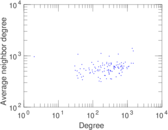### Zipf plot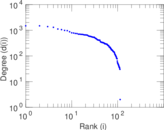### Hop distribution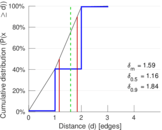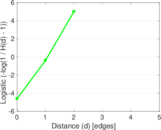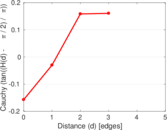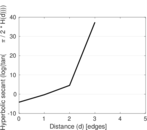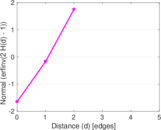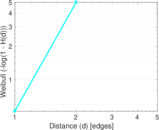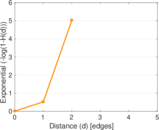### Double Laplacian graph drawing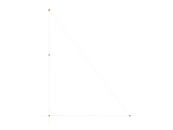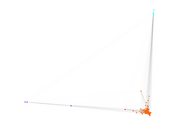### Delaunay graph drawing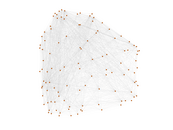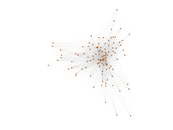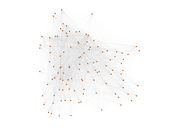### Edge weight/multiplicity distribution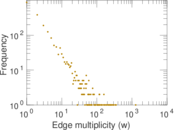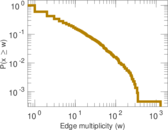### Clustering coefficient distribution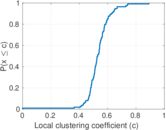### Average neighbor degree distribution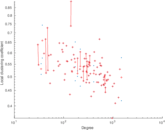### Temporal distribution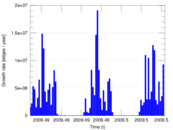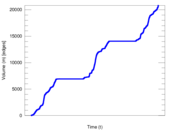### Temporal hop distribution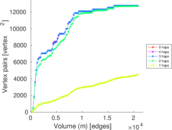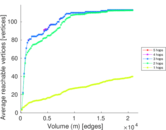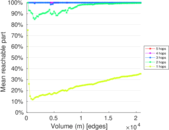### Diameter/density evolution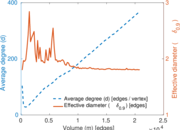### Inter-event distribution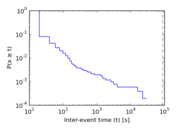### Node-level inter-event distribution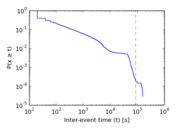### Matrix decompositions plots